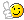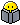Summarization Example Help!

we have 5 networks
172.1.4.128/25
172.1.7.0/24
172.1.6.0/24
172.1.5.0/24
172.1.4.0/25

How do you find the interesting octet here? In my book it says the third, however there is two network which is the 172.1.4.128/25 and 172.1.4.0/25 what about those one? I know that the other three are in the third octet but not that one that ends in 128 and the .4/25 one. The one in .128 is in the fourth octet as well as the 172.1.40/25. Also how do you find the block size? Usually i would do 256- the interesting octet of the subnet mask but in my book it says block size is 4. I assume because 4 is the lowest number in the ip address. Thanks guys!

• Posts: 14Member ■□□□□□□□□□
Hey,

So the book is right in saying the block size in third octet is 4. Because they all can be grouped together and summarized as 172.1.4.0/22 which would mean the third octet moves up in increments of 4. So first block would be 0 - 3 then the next block, which is the one you have listed is 4 to 7.

Hope that helps.
• Thanks for the reply. So the first block woudl be 0-3 than next block would be 4-7? I thought to find the block size you would have to use the mask? like the ones from 4-7 with a mask of /24 it would be 255.255.255.0 Than 256-256=1. So 1 would be the block size?
Tman87 wrote: »
Hey,

So the book is right in saying the block size in third octet is 4. Because they all can be grouped together and summarized as 172.1.4.0/22 which would mean the third octet moves up in increments of 4. So first block would be 0 - 3 then the next block, which is the one you have listed is 4 to 7.

Hope that helps.
• Let me put it like this for you to better understand. I cannot ask you to follow this, but will give you some edge until you master these. So as you see 172.1 remains constant and the rest keep changing. Since summarization help you achieve less advertisements you are going to do an address that fits everything under one hood. So in this case the third octet should catch your eye and as the previous post said yes the Block size is 4, since it keep all the addresses under one hood (4-7).

Block size and interesting octet are good. Now lets get across the subnet mask. So you need a a block size of 4 which require 2 bits and that is in the third octet. So the last two bits will be borrowed and the rest all are 1's in the third octet (11111100=252). So the subnet mask is 255.255.252.0.

Now the network address is 172.1.4.0 and the subnet mask is 255.255.252.0 (/22).
however there is two network which is the 172.1.4.128/25 and 172.1.4.0/25 what about those one?

Dude, that's the part of the address range. If you write 172.1.4.0/24 (172.1.4.0/25 + 172.1.4.128/25) both the groups will be a part of this one, instead the author wanted to give you more understanding and divided a single network into two by borowing a bit from hosts and and breaking it into two.

Practice Subnettingwhich is the only way you can be perfect All the best.2016 Certification Goals: CCNP Route /COLOR][B][/B][I][B]X[/B][/I][COLOR=#008000-->-->TShoot[], CCDP []
• Thank you! okay so looking at the third octet i found what the 5 networks had in common which was in the third octet 000001|xx. I notice instead of 0s you replaced the 0's with ones. and 111111|00. Can you do it either way? Thank you!
Nans wrote: »
Let me put it like this for you to better understand. I cannot ask you to follow this, but will give you some edge until you master these. So as you see 172.1 remains constant and the rest keep changing. Since summarization help you achieve less advertisements you are going to do an address that fits everything under one hood. So in this case the third octet should catch your eye and as the previous post said yes the Block size is 4, since it keep all the addresses under one hood (4-7).

Block size and interesting octet are good. Now lets get across the subnet mask. So you need a a block size of 4 which require 2 bits and that is in the third octet. So the last two bits will be borrowed and the rest all are 1's in the third octet (11111100=252). So the subnet mask is 255.255.252.0.

Now the network address is 172.1.4.0 and the subnet mask is 255.255.252.0 (/22).

Dude, that's the part of the address range. If you write 172.1.4.0/24 (172.1.4.0/25 + 172.1.4.128/25) both the groups will be a part of this one, instead the author wanted to give you more understanding and divided a single network into two by borowing a bit from hosts and and breaking it into two.

Practice Subnettingwhich is the only way you can be perfect All the best.• The 1's are for the subnet mask..... which make the device understand what the network part is and what the host past is in an address. Without making this complicated I would recommend you to go through the subnetting chapter again which be a revision and also will help you absorb more knowledge.
2016 Certification Goals: CCNP Route /COLOR][B][/B][I][B]X[/B][/I][COLOR=#008000-->-->TShoot[], CCDP []
• 0000.0100
0000.0111
0000.0110
0000.0101
0000.0100

thats all the hosts in binary but i see that in many places it overlooks importance if last matching bits are all 1 thus meaning to use that host bit to use as a marker which subnet summarization starts from since its borrowing two bits is 4th subnet that remains to start from,now not entirely correct on that but assume that it is overlooked in many instances thus someone using say 0.0/22 like they would if last matching bits were 0s.

If wrong please correct me.
• Fastest way here is going to take a look at the networks

172.1.4.128/25
172.1.7.0/24
172.1.6.0/24
172.1.5.0/24
172.1.4.0/25

since we start at 4 and we want to summarize up to 7 = we know that our block size is 4 and we count in the 3rd octet.
4 networks to be summarized - 256-4 = 252

172.1.4.0/22 or 255.255.252.0